## 5 Big Ideas Overview and Exemplification

#### Coherence

Coherence Teachers should develop detailed knowledge of the curriculum in order to break the mathematics down into small steps to develop mastery and address all aspects in a logical progression. Small steps are easier to take than big steps.

Procedural Variation – calculations are connected and pupils use the relationships to find easy ways of calculating.

Conceptual Variation – representing the same concepts in different ways to draw out essential features and form a fluent and deep understanding of the concept. Making connections between the different representations is important CPA all 3 are developed together – moving with fluency between the concrete and abstract.

Pupils represent concepts in different ways to demonstrate fluency and depth of understanding Representations expose mathematical structure which encourages children to work systematically.

Children and teachers need to think mathematically. This means to: look for pattern, make connections and reason about relationships.

Having instant recall of number bonds to ten and within ten and being able to apply these to multiples (eg 40 + 20 = 60 and bridging ten 8 + 5 = 8 + 2 + 3 = 13 is crucial for mastery of the curriculum Learn the facts so that the mind is freed up to think about concepts.

The five Big Ideas of teaching for mastery have been used to aid the development of teaching for mastery in UK classrooms. The five Big Ideas were identified from common aspects of effective teaching and learning and are used in the development of teaching for mastery work groups as a useful focus on learning and teaching.

# Partitioning Single Digit Numbers

Essential for 2 reasons:

– Prerequisite for addition by bridging through 10 (can’t solve 8 + 5 by making ten, unless you can partition 5 into 2 and 3)

– Prerequisite for fluency in subtraction facts – if you don’t just know that 9 partitions to 7 and 2 then the only way you will have to solve 9 – 7 is by counting back 9, 8, 7, 6, 5, 4, 3..

Use of a core structures eg part whole model, tens frame, numicon and then linking other structures and contexts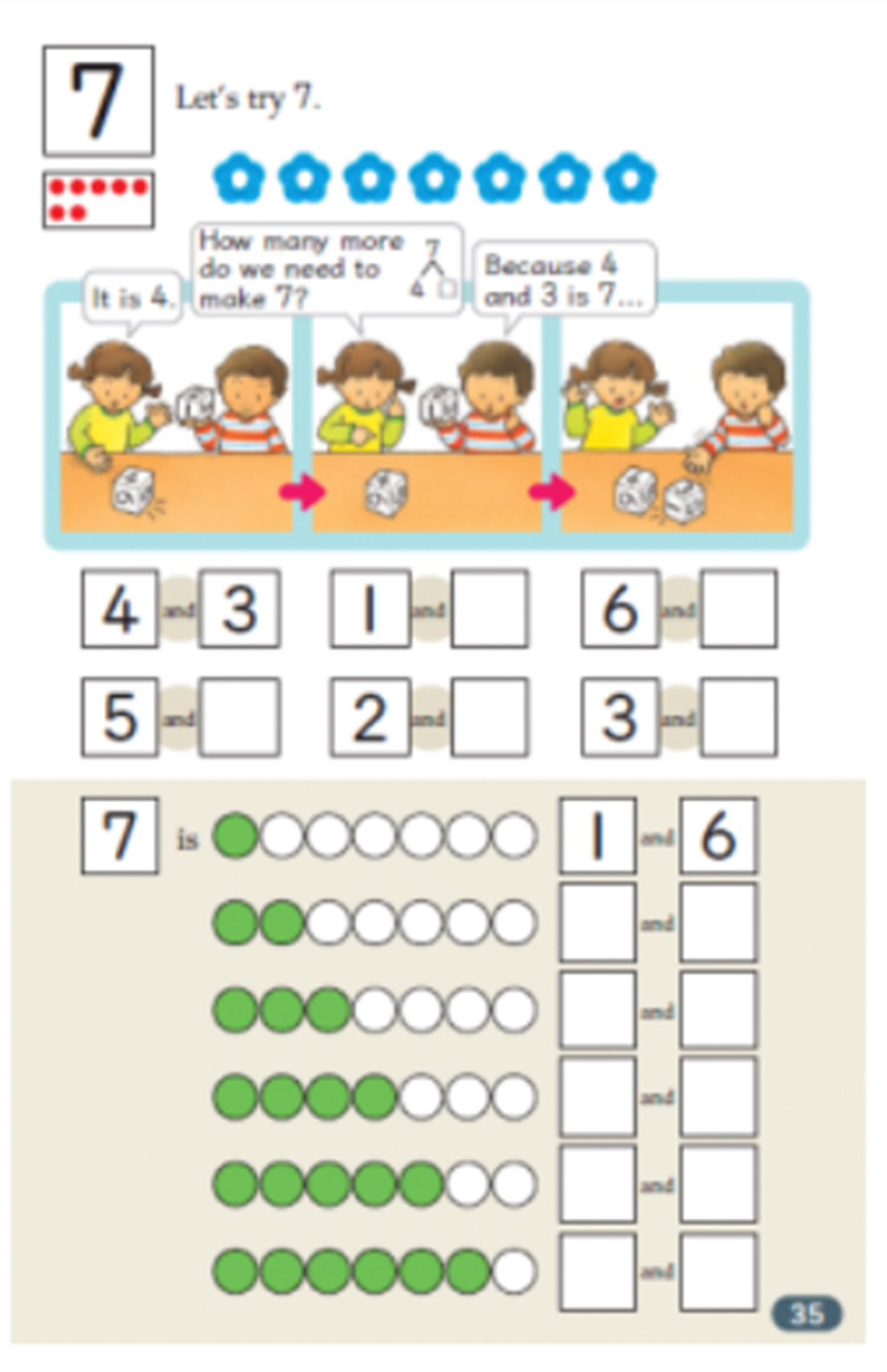Book 1 p35 Children learn to partition all numbers to 10.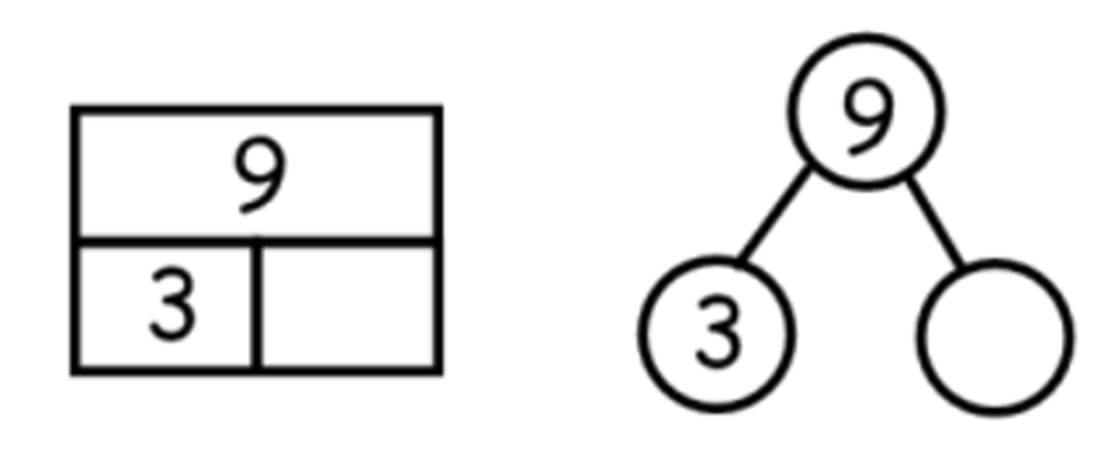Use of a core structure eg part whole model, tens frame, numicon and then linking other structures and contexts.

I can partition 7 in 6 different ways and still have 7.

I can partition 6 in 5 different ways.

I can partition 5 in 4 different ways.

Is there a pattern? Why does the pattern occur?

Knowing all the number bonds within 2, 3, 4, 5, 6 etc.

# Single Digit Number (SDN) Addition

– Addition facts which bridge 10

Here is a typical profile of a ‘fluent’ child at the end of Y3 (i.e. less than 3 seconds per fact) showing he has moved beyond counting for all facts.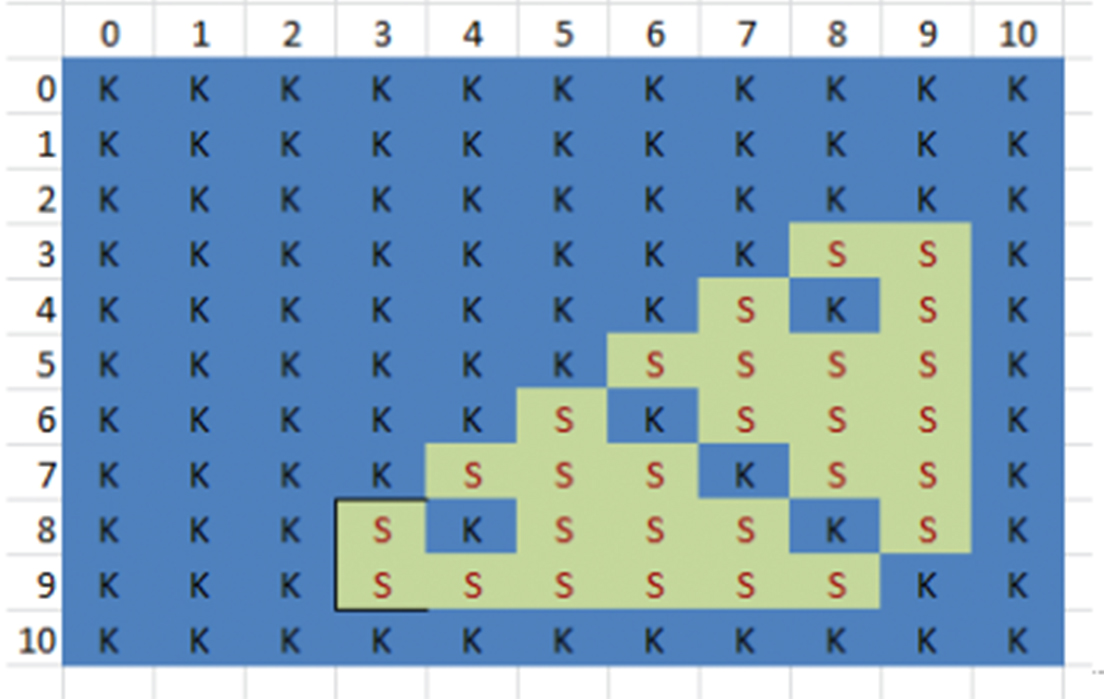And an end of Y2 child (not yet fluent)

(K=known, S=strategy, C=counting)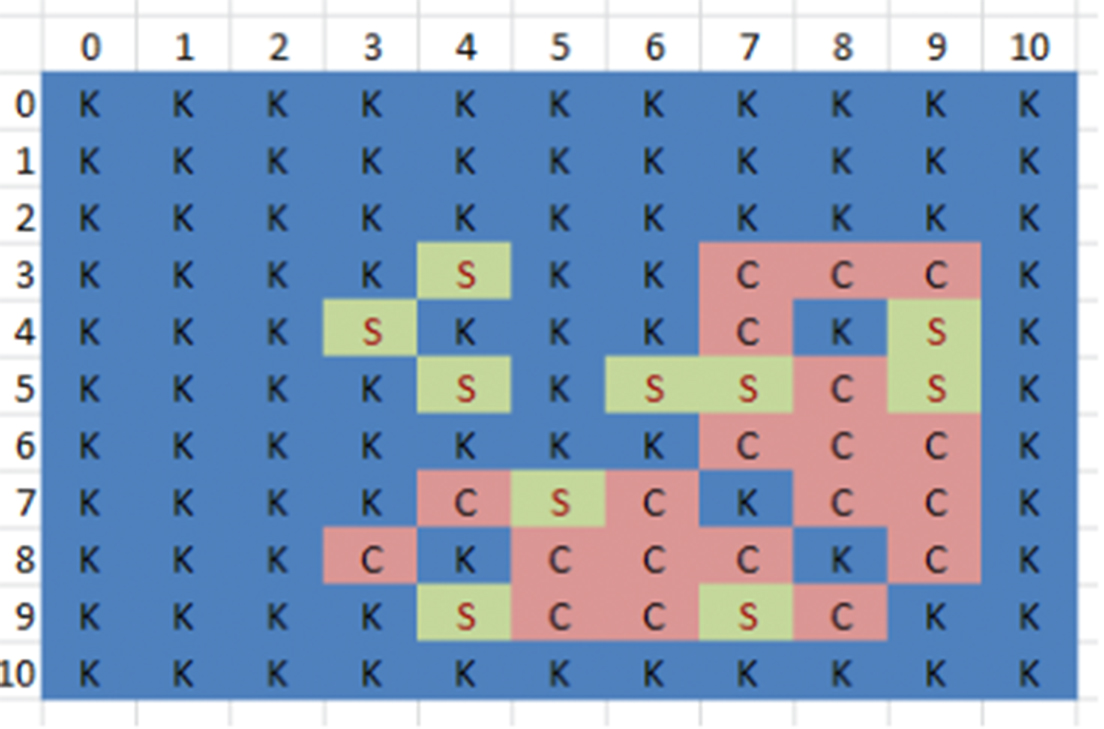Variation of unit: 3 + 4 = 7 3 tens + 4 tens = 7 tens (so 30 + 40 = 70)

3 million + 4 million = 7 million 3 cats + 4 cats = 7 cats

£3 + £4 = £7 38 + 48 = 78

3 tenths + 4 tenths = 7 tenths (so 0.3 + 0.4 = 0.7)

3km + 4km = 7km 3 groups of 5 + 4 groups of 5 = 7 groups of 5 (so 3×5 + 4×5= 7×5 or distributive law of multiplication)

3x + 4x = 7x

Use of ‘make 10’ to work with addends of 7, 8 and 9. Models first e.g. 9 + 5, 8 + 3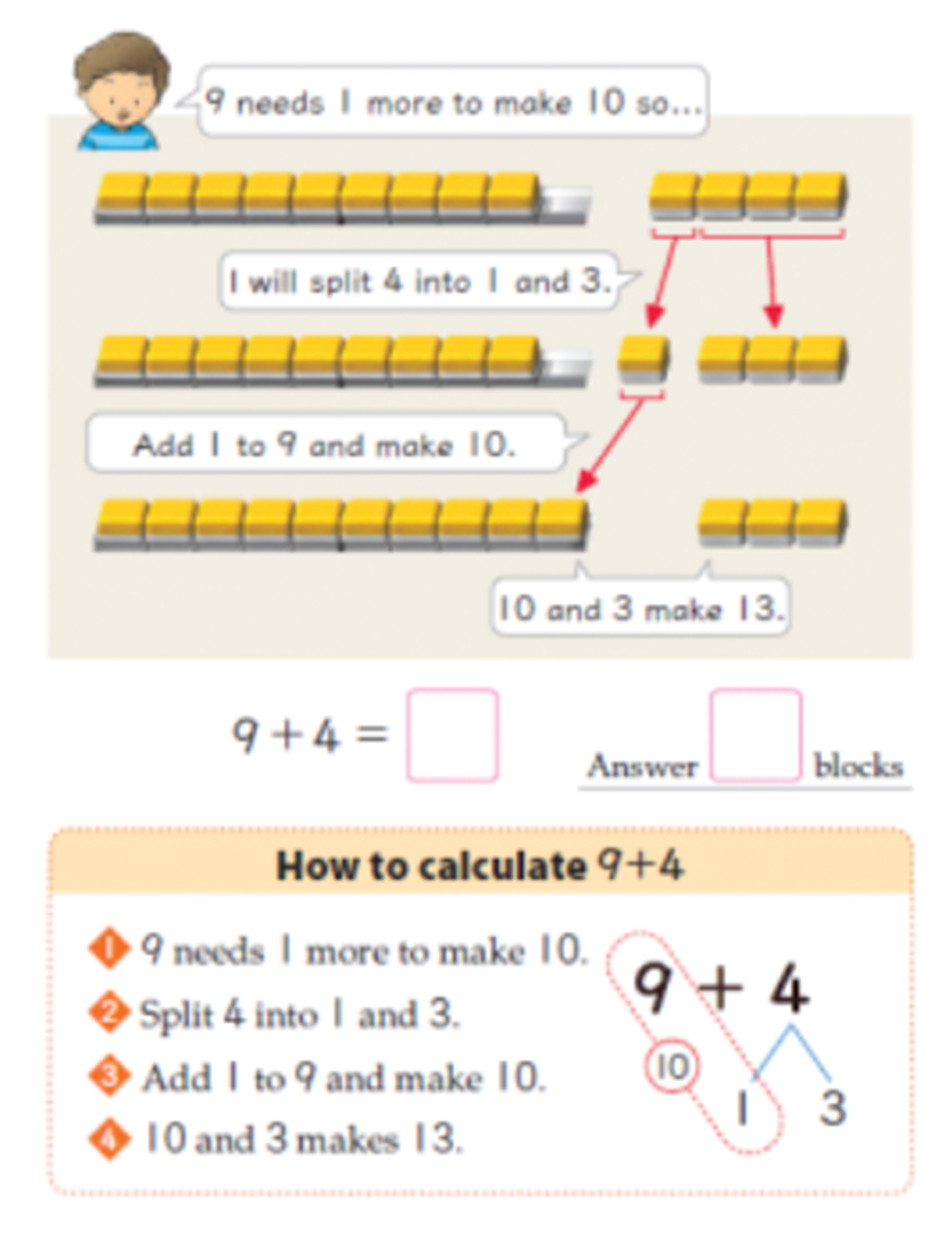…then moves onto e.g. 3 + 9, 4 + 8I know 7 + 3 = 10, so 10 – 3 must equal 7 and 7 + 4 must equal 11 – a chain of reasoning based on mathematical relationships.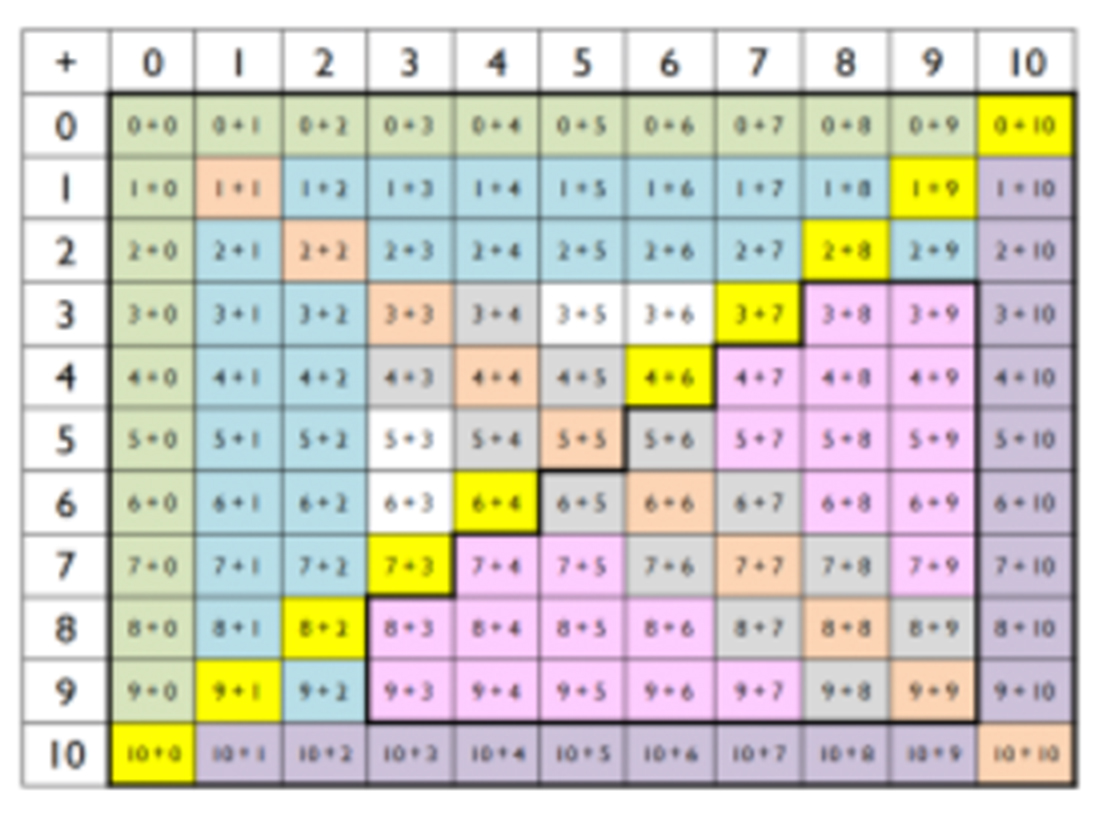Is the approach to every single SDN+ SDN calculation taught in your school?

E.g. do you explicitly teach the children that SDN + 2 involves moving from odd to the next odd number, or even to the next even number? So a building block for this is fluent counting in odds and evens.

No children should be thinking 7, 8, 9 – just 7, 9

# Subtraction within 10 Subtraction bridging 10

Subtraction structures of partitioning, take away and difference The relationship between addition and subtraction.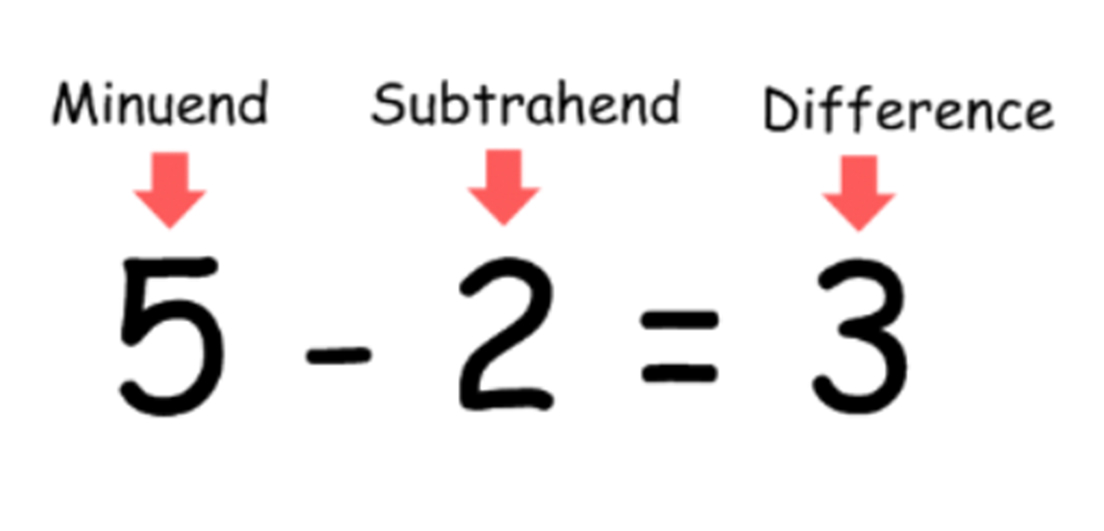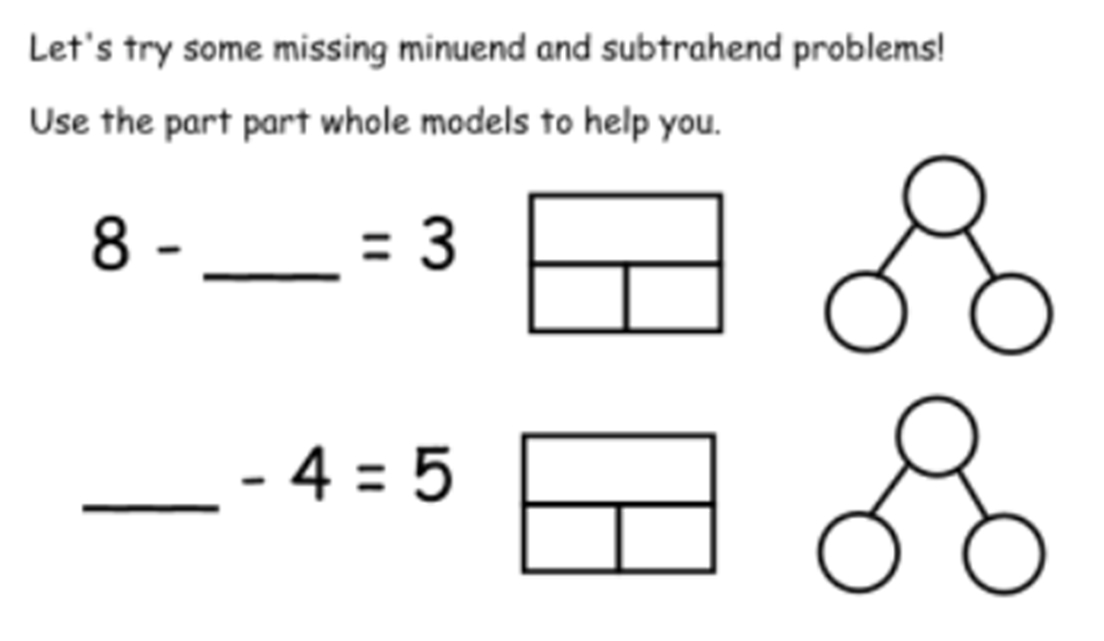Partitioning single digit numbers as an essential precursor to subtraction within 10…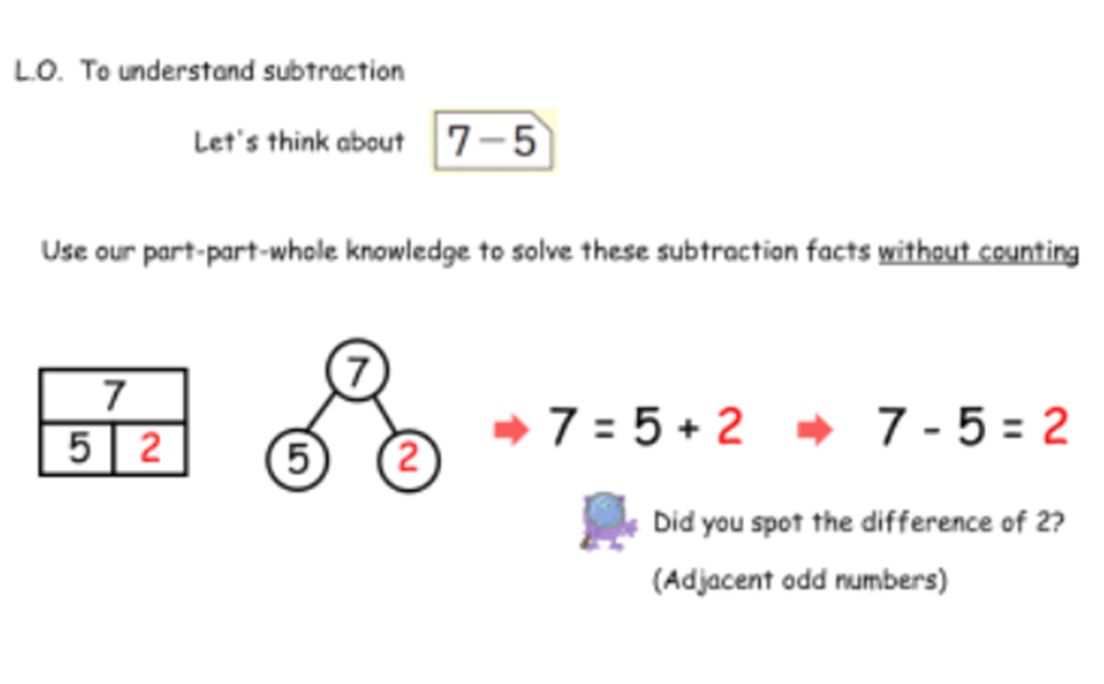Modelling difference: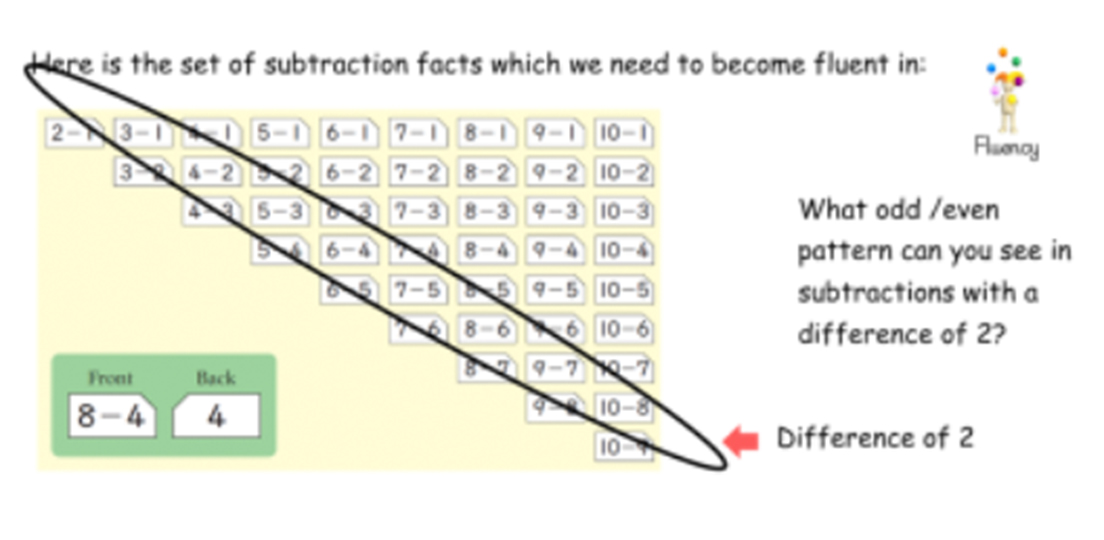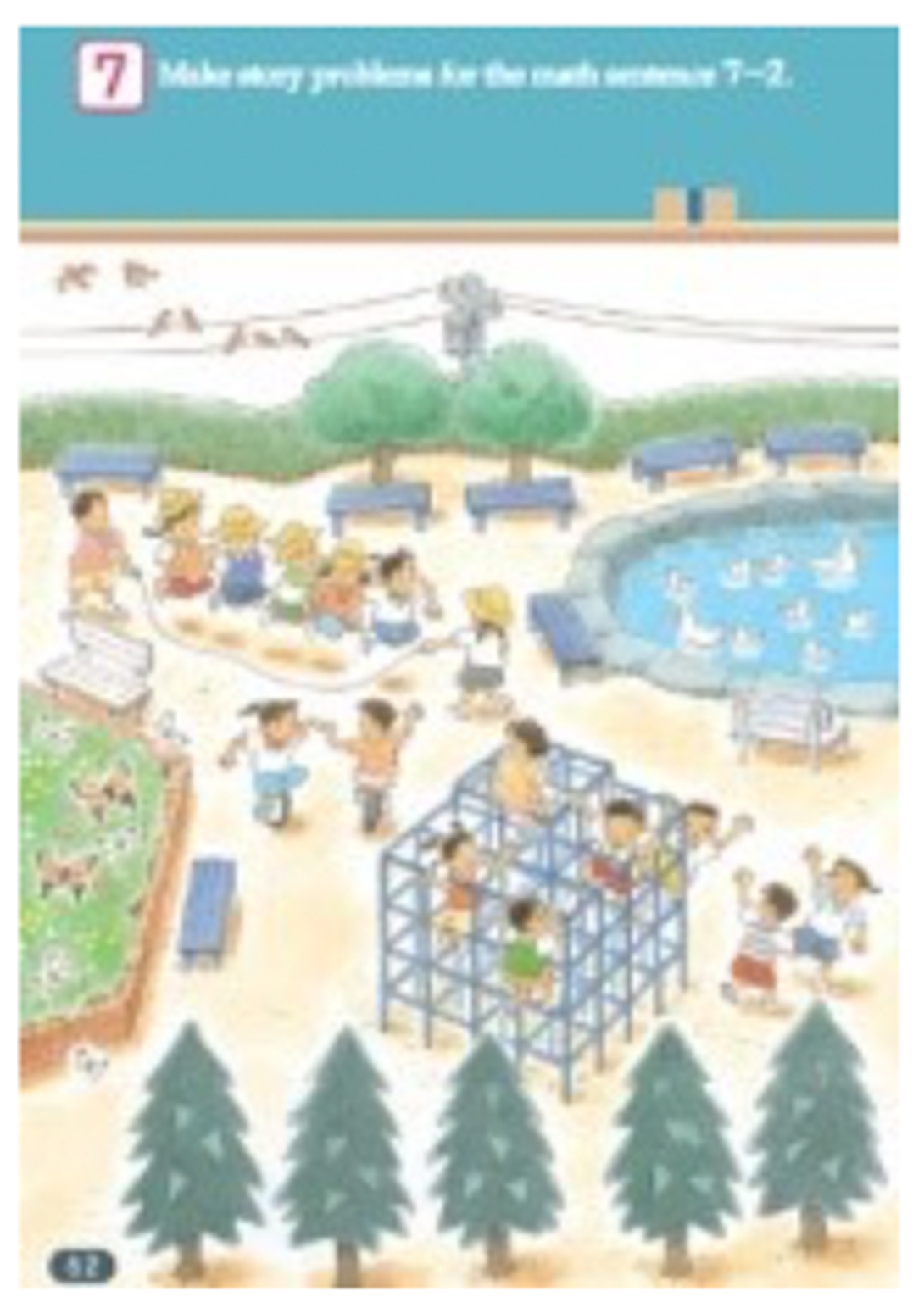Can you write story problems from the pic for 7

– 2 for all 3 subtraction situations:

+ Partitioning

+ Take away

+ Difference

This involves moving between the concrete and abstract.

Story of 13’ – moving from partitioning to fluency in subtraction facts which bridge 10.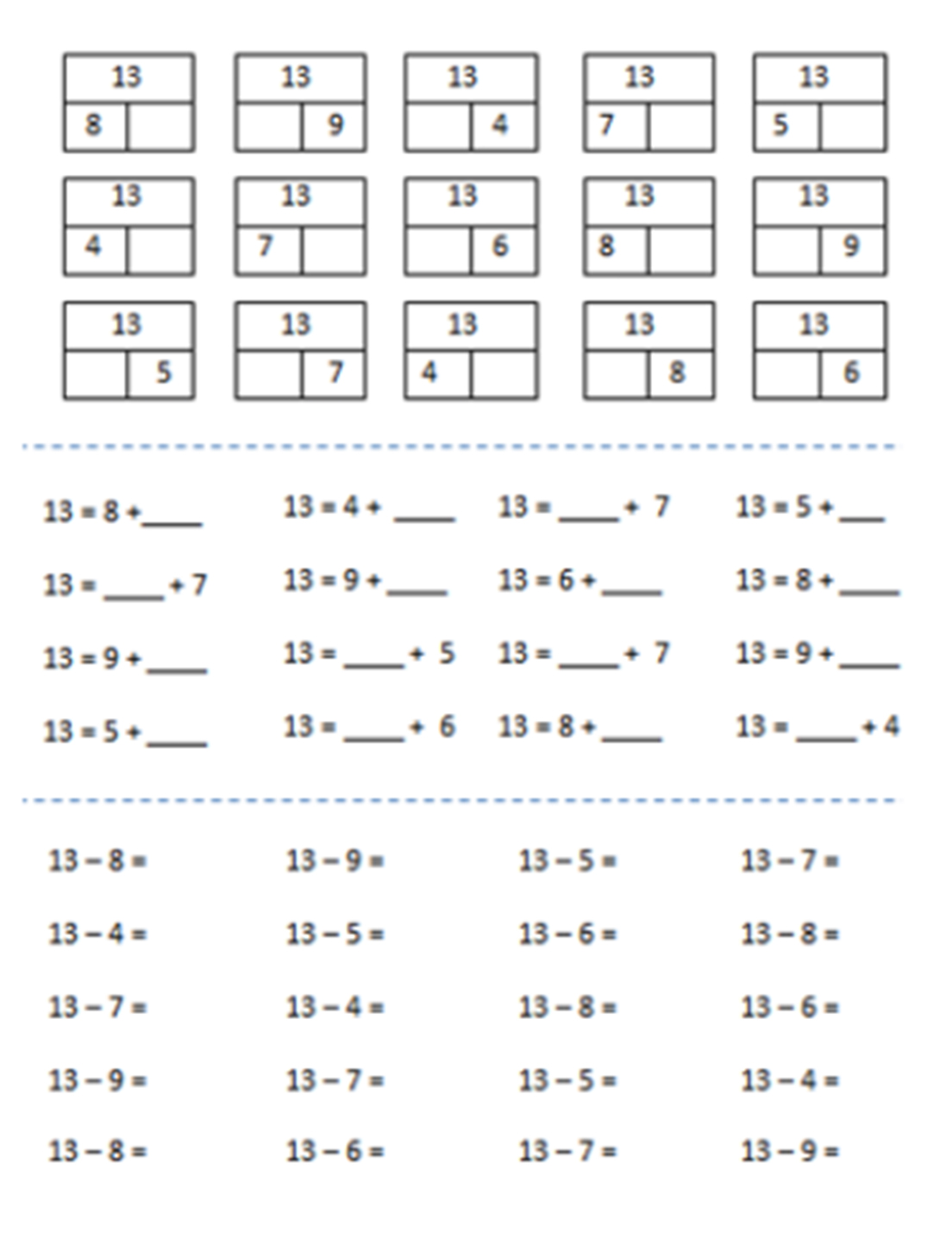Complete focus on a clear number fact set, which children become fluent in. The children are shown ‘these are the facts we are using and learning in this subtraction unit’.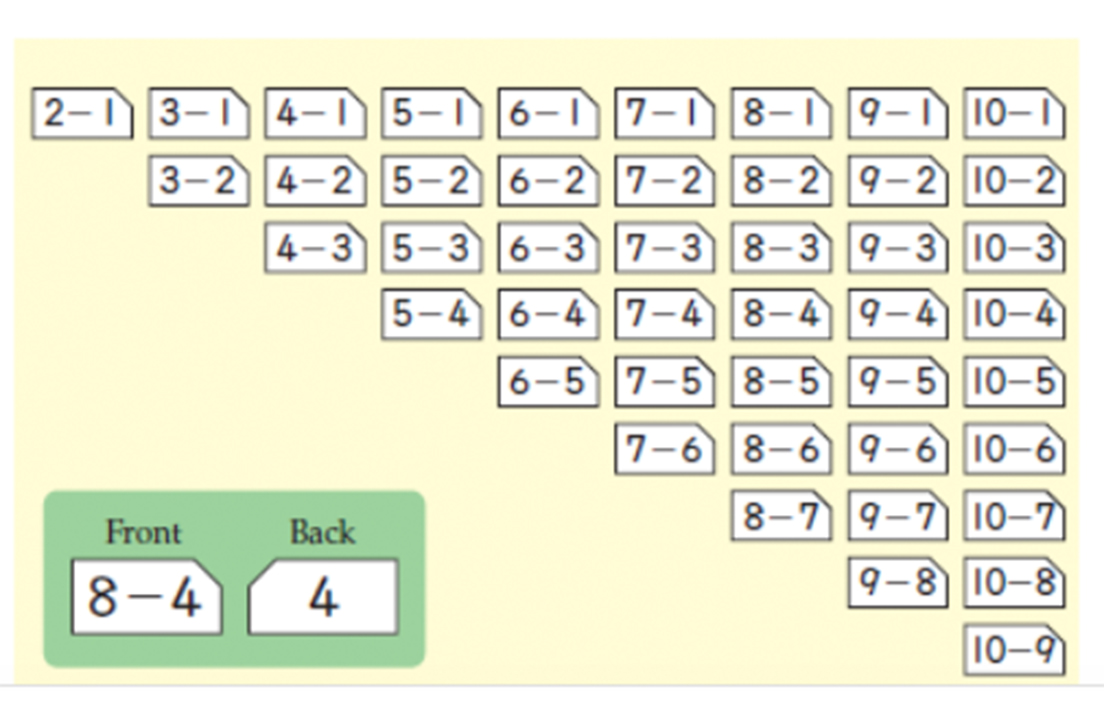Book 1, p56

Very clear that subtraction facts to be learnt include e.g. 13 – 5 but not 15 – 3, which is just a place value extension of 5 – 3 (already learnt) rather than a ‘new’ fact.

There is an important structural difference between 13 – 5 and 15 -3, so don’t just lump them all into ‘subtraction within 20’.

Here are the new facts: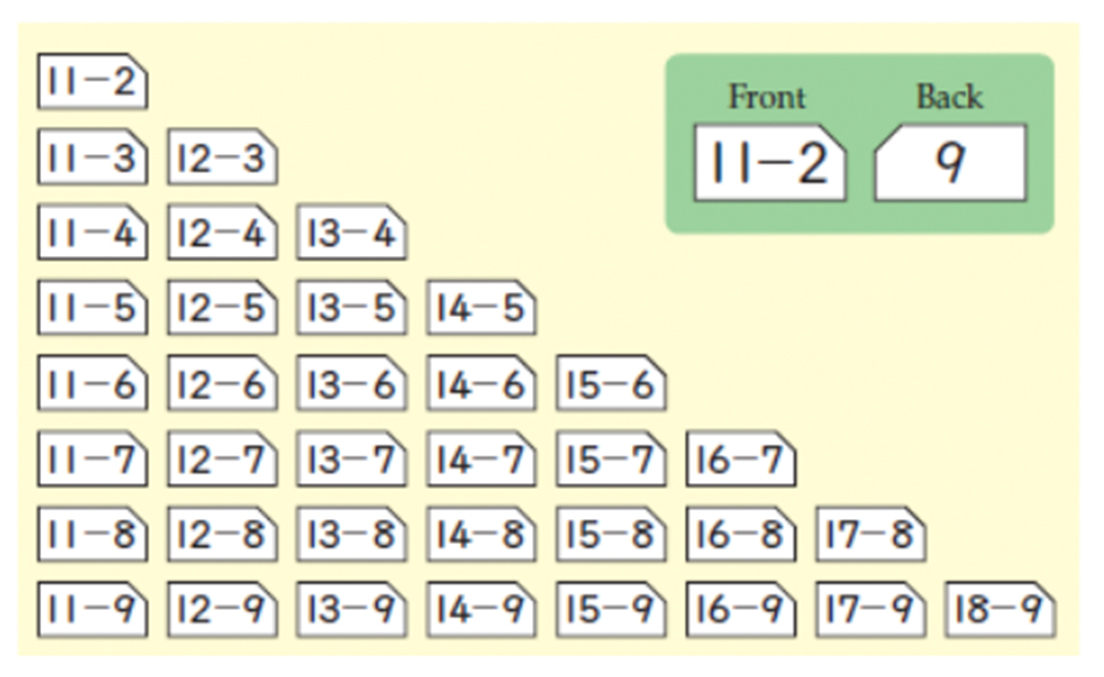Book 1, p112

# Working with equations with more than 2 e.g. 5 + 4 + 2

Working with equations with more than 2 terms

Working with mixed operations 3 – 1 + 10

5 + 4 + 2 = 11

4 + 5 + 2 = 11

3 + 6 + 2 = 11

2 + 7 + 2 = 11

1 + 8 + 2 = 11

What do you notice?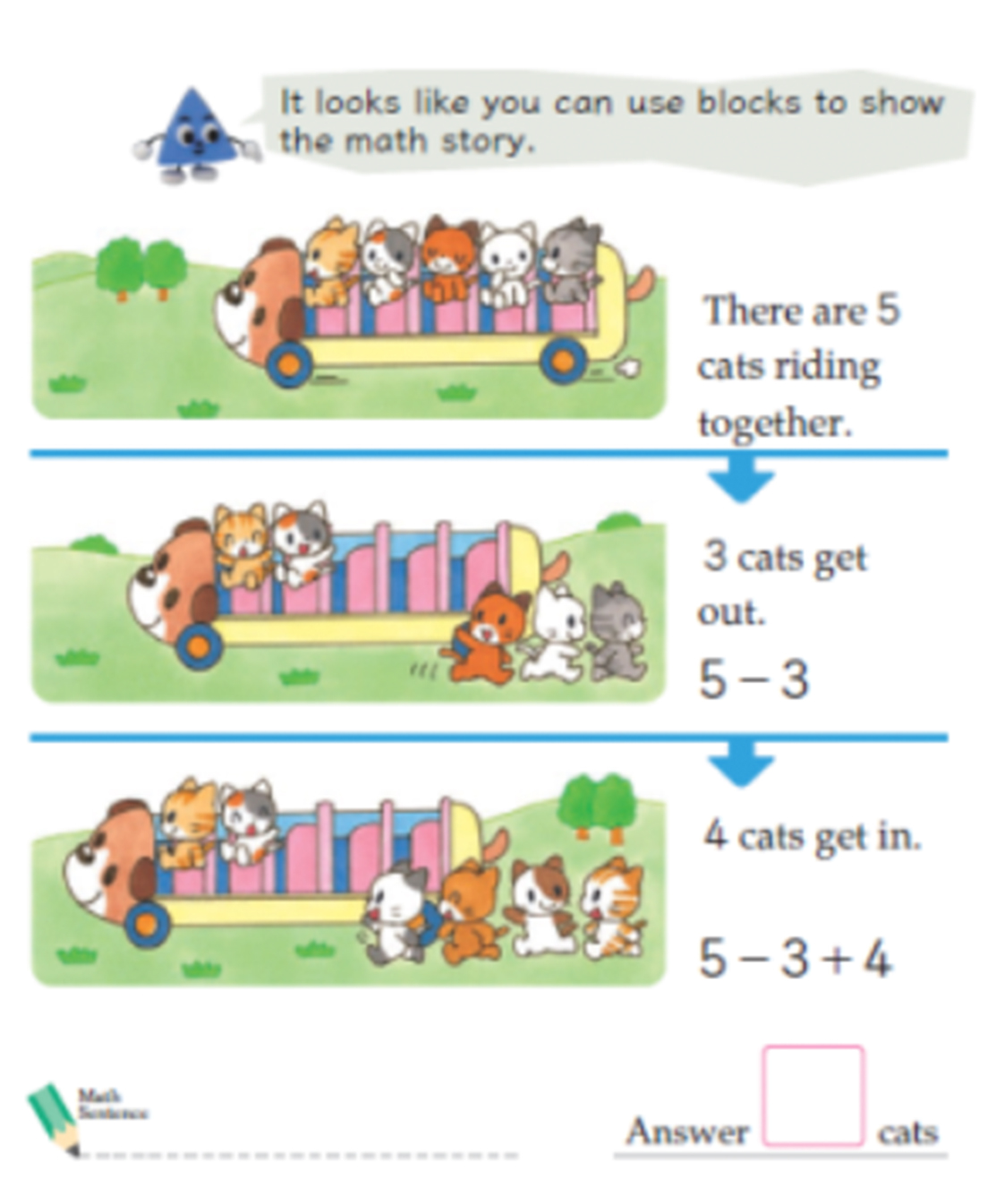Reasoning about relationships using empty box problems.

Redistributing: 999 + 99 + 9 + 3 = 1000+ 100 + 10

Re balancing: 34 + ____ = 58 + 44

Same difference: 82 – 65 = 78 – ____

Fluency in moving between the abstract and concrete representations.

# Extending addition and subtraction facts with place value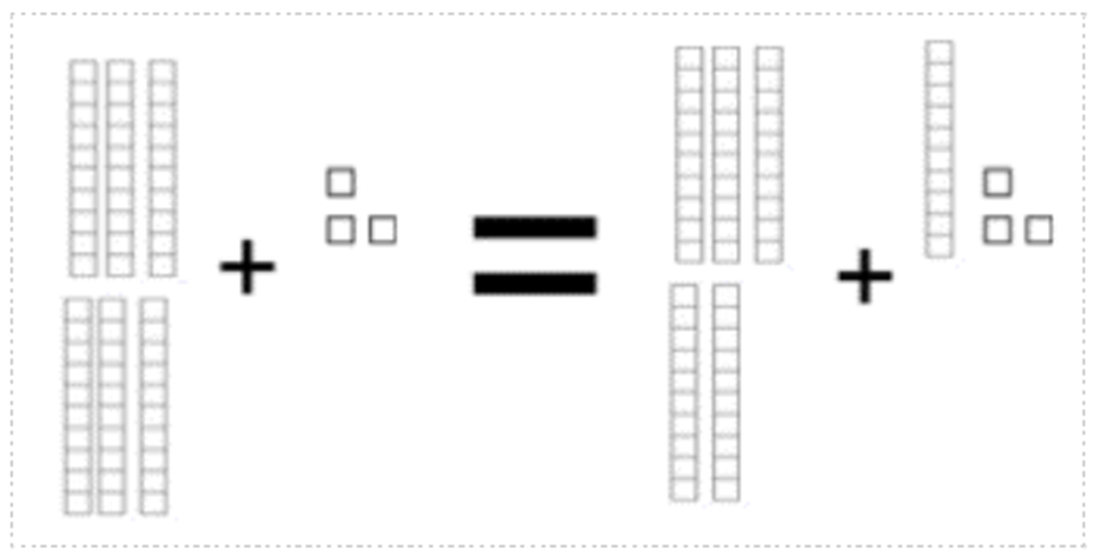[Continue the pattern using the dienes for support if needed]

SO 50 + 35 = ____ + 45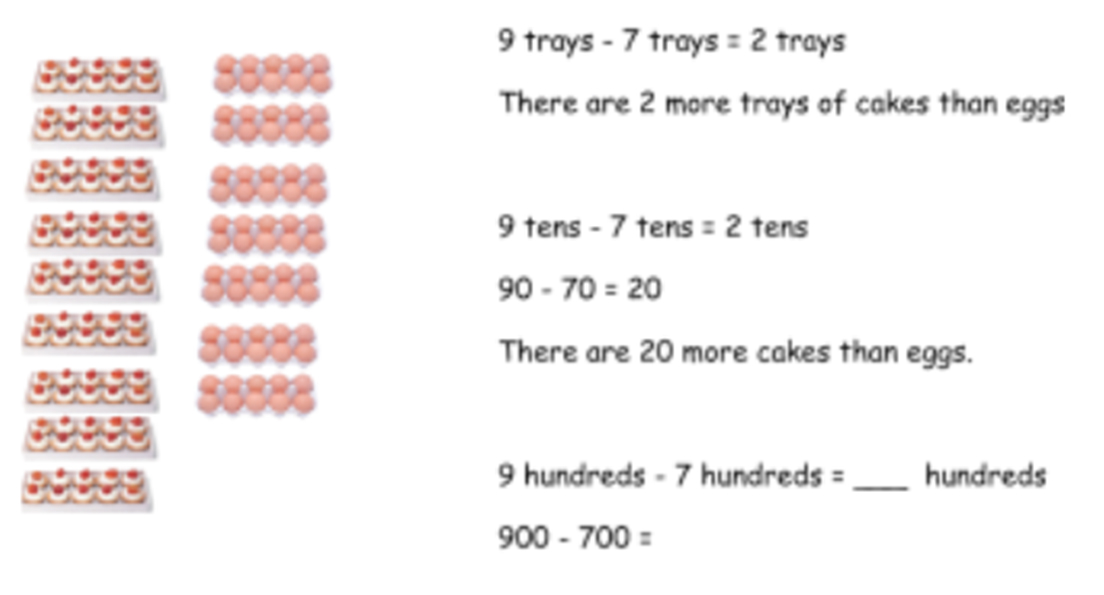Redistributing: 999 + 99 + 9 + 3 = _____

Re balancing: 34 + ____ = 58 + 44

Same difference: 82 – 65 = 78 – ____

2 + 4 = 6

Therefore 20 + 40 = 60

Putting the spotlight on difference.

Can you find pairs of numbers with a difference of 2?

How about a difference of 10?

34 – 24 = 10 71 – 61 = 10 50 – 60 = 10

This approach can obviously lead into modelling the same difference problems like 82 – 65 = 78 – ____

Fluency with both single digits and multiples.